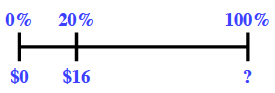### Home > CC2MN > Chapter 7 > Lesson 7.2.6 > Problem7-125

7-125.Alex has a job delivering newspapers. He puts $20\%$ of his earnings each week into his college savings account. Each week he puts $\16$ into the account.

1. Draw a diagram to represent this situation. How much money does Alex earn each week?$\16$ is $20\%$ of what?

$\frac{20}{100}=\frac{16}{\textit{x}}$

$\80$

2. Alex spends $10\%$ of his earnings on snacks each week. How much does he spend?

Find $10\%$ of the answer from part (a).

$0.10(80)$

$\8$

3. When Alex has worked for one year, he will get a raise that is equal to $15\%$ of his current earnings. How much more money will he earn each week?

Find $15\%$ of earnings per week, the answer from part (a).